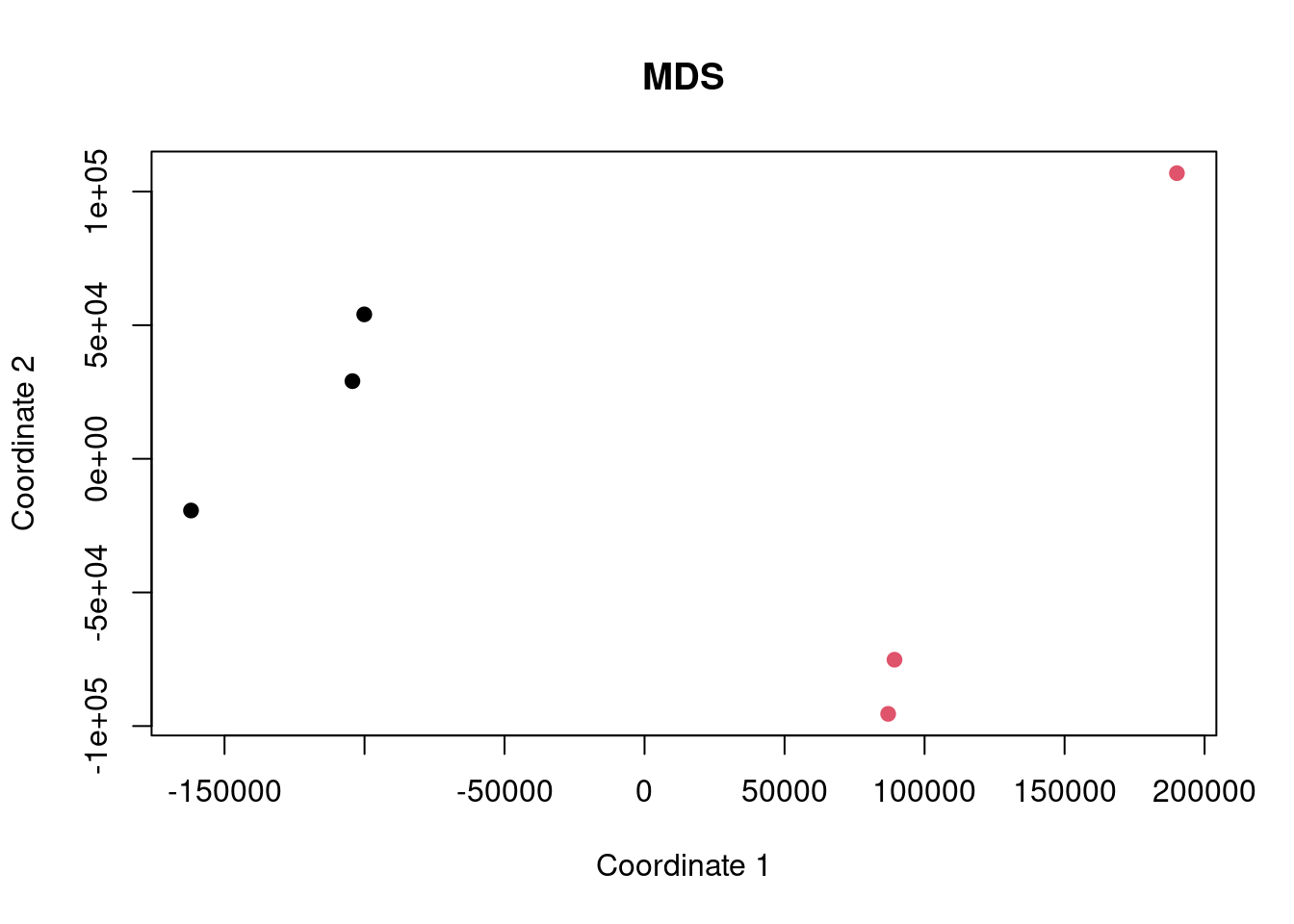## Intro

Here we are performing an analysis of some gene expression data to demonstrate the difference between ORA and FCS methods and to highlight the differences caused by improper background gene set use.

The dataset being used is SRP068733 and we are comparing the healthy endothelial cells with a scrambled siRNA to cells treated with a p300 targeting siRNA.

Data are obtained from http://dee2.io/

``````suppressPackageStartupMessages({
library("getDEE2")
library("DESeq2")
library("clusterProfiler")
library("mitch")
library("kableExtra")
library("eulerr")
})``````

## Get expression data

I’m using some RNA-seq data looking at the difference in healthy endothelial cell gene expression between vehicle and C646 samples.

``````name="SRP068733"
samplesheet <- mdat[grep("SRP068733",mdat\$SRP_accession),]
samplesheet<-samplesheet[order(samplesheet\$SRR_accession),]
SRRvec <- c("SRR3112216","SRR3112217","SRR3112218","SRR3112219","SRR3112220","SRR3112221")
samplesheet <- samplesheet[which(samplesheet\$SRR_accession %in% SRRvec),]
samplesheet\$trt<-as.factor(c(0,0,0,1,1,1))
s1 <- samplesheet

s1 %>% kbl(caption = "sample sheet") %>% kable_paper("hover", full_width = F)``````
sample sheet
SRR_accession QC_summary SRX_accession SRS_accession SRP_accession Sample_name GEO_series Library_name trt
299400 SRR3112216 PASS SRX1540348 SRS1256815 SRP068733 GSM2044428 GSE77108 0
299401 SRR3112217 PASS SRX1540349 SRS1256814 SRP068733 GSM2044429 GSE77108 0
299402 SRR3112218 PASS SRX1540350 SRS1256812 SRP068733 GSM2044430 GSE77108 0
299403 SRR3112219 PASS SRX1540351 SRS1256813 SRP068733 GSM2044431 GSE77108 1
299404 SRR3112220 PASS SRX1540352 SRS1256811 SRP068733 GSM2044432 GSE77108 1
299405 SRR3112221 PASS SRX1540353 SRS1256810 SRP068733 GSM2044433 GSE77108 1
``w<-getDEE2("hsapiens",SRRvec,metadata=mdat,legacy = TRUE)``
``````## For more information about DEE2 QC metrics, visit
##     https://github.com/markziemann/dee2/blob/master/qc/qc_metrics.md``````
``````x<-Tx2Gene(w)
x<-x\$Tx2Gene

# save the genetable for later
gt<-w\$GeneInfo[,1,drop=FALSE]
gt\$accession<-rownames(gt)

# counts
x1<-x[,which(colnames(x) %in% samplesheet\$SRR_accession)]``````

Here show the number of genes in the annotation set, and those detected above the detection threshold.

``````# filter out lowly expressed genes
x1<-x1[which(rowSums(x1)/ncol(x1)>=(10)),]
nrow(x)``````
``##  39297``
``nrow(x1)``
``##  14255``

Now multidimensional scaling (MDS) plot to show the correlation between the datasets. If the control and case datasets are clustered separately, then it is likely that there will be many differentially expressed genes with FDR<0.05.

``plot(cmdscale(dist(t(x1))), xlab="Coordinate 1", ylab="Coordinate 2", pch=19, col=s1\$trt, main="MDS")``## Differential expression

Now run DESeq2 for control vs case.

``y <- DESeqDataSetFromMatrix(countData = round(x1), colData = s1, design = ~ trt)``
``## converting counts to integer mode``
``y <- DESeq(y)``
``## estimating size factors``
``## estimating dispersions``
``## gene-wise dispersion estimates``
``## mean-dispersion relationship``
``## final dispersion estimates``
``## fitting model and testing``
``````de <- results(y)
de<-as.data.frame(de[order(de\$pvalue),])
rownames(de)<-sapply(strsplit(rownames(de),"\\."),"[[",1)
head(de) %>% kbl() %>% kable_paper("hover", full_width = F)``````
baseMean log2FoldChange lfcSE stat pvalue padj
ENSG00000049449 10375.678 -1.319179 0.0346992 -38.01753 0 0
ENSG00000065308 9062.133 -1.354569 0.0335259 -40.40362 0 0
ENSG00000066056 10429.917 -1.273349 0.0333485 -38.18314 0 0
ENSG00000068001 6526.375 -1.635372 0.0423455 -38.61975 0 0
ENSG00000076706 25433.097 -1.625573 0.0301945 -53.83679 0 0
ENSG00000087245 19077.593 -1.541325 0.0312561 -49.31281 0 0

Now let’s have a look at some of the charts showing differential expression. In particular, an MA plot and volcano plot.

``````maplot <- function(de,contrast_name) {
sig <-subset(de, padj < 0.05 )
up <-rownames(subset(de, padj < 0.05 & log2FoldChange > 0))
dn <-rownames(subset(de, padj < 0.05 & log2FoldChange < 0))
GENESUP <- length(up)
GENESDN <- length(dn)
DET=nrow(de)
SUBHEADER = paste(GENESUP, "up, ", GENESDN, "down", DET, "detected")
ns <-subset(de, padj > 0.05 )
plot(log2(de\$baseMean),de\$log2FoldChange,
xlab="log2 basemean", ylab="log2 foldchange",
pch=19, cex=0.5, col="dark gray",
main=contrast_name, cex.main=0.7)
points(log2(sig\$baseMean),sig\$log2FoldChange,
pch=19, cex=0.5, col="red")
}

make_volcano <- function(de,name) {